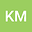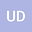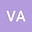THE SOLUTIONS OF A CONFORMABLE TIME FRACTIONAL NLPDE VIA IBSEFM
•••• Khanlar Mamedov,
• Ulviye Demirbilek,
• Volkan Ala
Khanlar Mamedov
Mersin Universitesi
Author ProfileUlviye Demirbilek
Mersin Universitesi
Author ProfileVolkan Ala
Mersin Universitesi
Author Profile## Abstract

The nonlinear conformable time-fractional the simplified modified Camassa-Holm (MCH) equation plays an important role in physics. It is an interesting model to define change waves with weak nonlinearity. In this paper, the space-time fractional derivatives are defined in the sense of the new conformable fractional derivative. The new exact solutions of MCH equation using Improved Bernoulli Sub-Equation Function Method (IBSEFM) are constructed, the 2D graphs and 3D graphs acquired from the values of the solutions are given.The results show that IBSEFM is a powerful mathematical tool to solve nonlinear conformable time-fractional partial differantial equations (CTFPDEs) arising in mathematical physics.